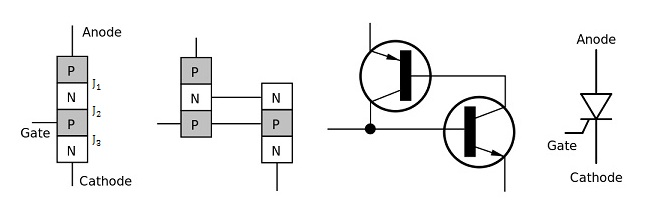# Difference Between Transistor and Thyristor

## Main Difference – Transistor vs. Thyristor

Transistors and thyristors are both semiconductor devices which have numerous applications in electric circuits. The main difference between transistor and thyristor is that a transistor has three layers of semiconductors, whereas a thyristor has four layers of semiconductors. Sometimes, thyristors are referred to as silicon controlled rectifiers (SCRs).

## What is a Transistor

Transistors are semiconductor devices that can act as amplifiers or switches in electric circuits. A transistor consists of three doped semiconductors. Major types of transistors include bipolar junction transistors (BJTs) and field effect transistors (FETs) and insulated gate bipolar transistors (IGBTs). We have discussed how these transistors work in the articles comparing the difference between BJTs and FETs and the difference between IGBTs and MOSFETs. Transistors have three terminals. By controlling the voltage applied to one of the terminals, it is possible to control the current through the other two terminals of these devices.

## What is a Thyristor

A thyristor also has three terminals like a transistor, and these terminals are called “anode”, “cathode” and “gate”. However, a thyristor is made of four layers of doped semiconductors. Functionally, a thyristor acts like a combination of two transistors, as depicted below:You can think of a thyristor as two transistors working together. On the right: the symbol for a thyristor.

A thyristor has three modes:

1. Reverse blocking mode: In this setup, the anode is given a more negative potential than the cathode. This means that the junctions J1 and J3 are reverse biased while the junction J2 is forward biased. In this mode, a current cannot flow through the thyristor.
2. Forward blocking mode: In this setup, the anode is given a more positive potential than the cathode. Here, J1 and J3 are forward biased, while J2 is in reverse bias. A current still cannot flow through the thyristor.
3. Forward conducting mode: In this setup, the anode and the cathode are connected as in the forward blocking mode. However, there is now a current flowing through the thyristor. This could have been achieved by two methods: If the forward potential difference between the anode and cathode was so large, the junction J2 would undergo breakdown, allowing a current to flow across it. If the potential difference is not large enough for breakdown to occur, forward conduction could also have been achieved by sending a forward current through the gate.

If a current is applied at the gate and the forward current in the thyristor reaches a threshold value of current known as the latching current, the thyristor would continue to conduct even when the gate current is removed. Once the thyristor has started conducting a forward current, it can continue to do so, for as long as the forward current is above a threshold current value known as the holding current. For this reason, the thyristor can be used as a switch. The figure below shows the current vs. voltage characteristic for a thyristor:The current vs. voltage characteristic curve for a thyristor.

The curve labelled$I_G=0$ refers to the case when there is no applied gate current. Here, the forward voltage must reach the breakdown value of$V_{BO}$ before it can start to conduct a significant current. The curve labelled$I_G>0$ shows that when there is some gate current, a current can start flowing through the thyristor at a lower forward voltage. Note that the current values labelled$I_L$ and$I_H$ refer to the latching current and holding current respectively. The curves show that once latching current is achieved, the current rises rapidly and that if the current falls to$I_H$, it drops off (the dashed curve).

## What is the difference between Transistor and Thyristor

### Number of Semiconductor Layers

Transistors consist of three layers of semiconductors.

Thyristors consist of four layers of semiconductors.

### Power Ratings

Thyristors can be used in circuits delivering larger amounts of power compared to transistors.

### Usage as an Amplifier

Transistors can be used as switches or amplifiers.

Thyristors can be used as switches, but not as amplifiers.

### Maintaining a Forward Current

In transistors, a continuous input is required to maintain a forward current.

In thyristors, a pulse can get the forward current flowing, and this current will continue to flow as long as it does not fall below a threshold value, even when there is no longer an input current.

Image Courtesy:

“Diagram of a thyristor” by Riflemann~commonswiki (Own work) [CC BY-SA 3.0], via Wikimedia Commons (Modified)

“Thyristor current–voltage characteristic” by Mikhail Ryazanov (Own work) [Public Domain], via Wikimedia Commons